# Multiplication Trick for Large Numbers

Today we are sharing some special cases of multiplication which will help you to solve simplification problems quickly and easily. Try to apply these techniques for speedy calculations and keep practicing !!

I have already shared Vedic maths trick to Multiply large numbers here.

### GENERAL RULES FOR MULTIPLICATION

#### MULTIPLICATION BY A 2-DIGIT NUMBER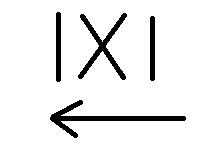EXAMPLE - 62 × 71
Step 1 - Multiply the right hand side digitsStep 2 - Cross-MultiplicationStep 3 - Multiply the left hand side digits#### MULTIPLICATION BY A 3-DIGIT NUMBEREXAMPLE - 198 × 256

Step 1 -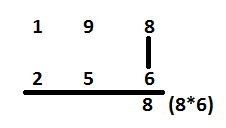Step 2-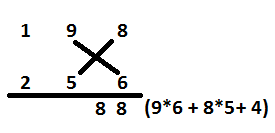Step 3 -Step 4 -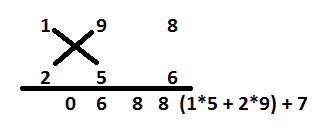Step 5 -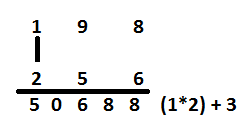#### MULTIPLICATION BY A 4-DIGIT NUMBER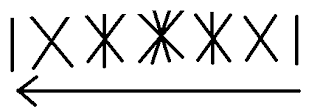EXAMPLE -  4325 × 3216

Step 1 -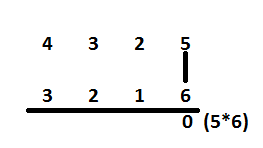Step 2 -Step 3 -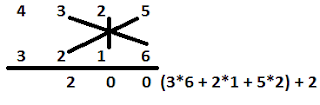Step 4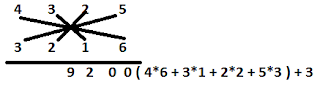Step 5Step 6Step 7#### Multiply by 5

To multiply any number with 5, we will

"MULTIPLY THE NUMBER WITH 10 AND DIVIDE IT  WITH 2  "

EXAMPLE

Multiply 2469 by 5

Step 1 - Multiply 2469 with 10
=> 2469 ×  10 = 24690

Step 2  - Divide 24690 by 2
=> 24690/2 = 12345

#### MULTIPLY BY 9

To multiply any number with 9, we will

" MULTIPLY THE NUMBER WITH 10 AND SUBTRACT THE NUMBER ITSELF "

EXAMPLE

Multiply 4582 by 9

Step 1 - Multiply 4582 with 10
=> 4582 × 10 = 45820

Step 2 -Subtract the number itself from the result
=> 45820 - 4582 = 41238

#### MULTIPLY BY 11

To multiply any number with 11,we will

" WRITE ZERO TO THE LEFT SIDE OF THE NUMBER. KEEP THE LAST DIGIT SAME AND START ADDING THE SUCCESSIVE DIGITS FROM RIGHT HAND SIDE "

OR

" MULTIPLY THE NUMBER WITH 10 AND ADD THE NUMBER ITSELF "

EXAMPLE

Multiply 89067 by 11

Step 1 - Write zero to the left side of the number.
=> 089067

Step 2 - Keep the last digit same and start adding the digits from right hand side.

=>OR
Step 1 - Multiply the number with 10
=> 89067 × 10 = 890670

Step 2 - Add the number itself to the result
=> 890670 + 89067 = 979737

#### MULTIPLY BY 25

To multiply any number with 25, we will

" MULTIPLY THE NUMBER WITH 100 AND DIVIDE IT WITH 4 "

EXAMPLE

#### MULTIPLY 15698 by 25

Step 1 - Multiply the number with 100
=> 15698*100 = 156980

Step 2- Divide it with 4
=> 1569800/4 = 392450

#### MULTIPLICATION OF NUMBER CLOSE TO 100,1000 ...

Step 1 - Take base as 100 and find the distance of the numbers from 100.
Step 2 - Add the distances of the numbers by considering signs of the numbers and add the result to 100 and put two zeros to the right side of the number
Step 3 - Now, Multiply the distances of the numbers and add to the result of step 2.
Step 4 - Add the values of Step 3 and Step 4.

Lets understand this technique with the help of example :

Multiply 92 ×  97In case of 1000, take base as 1000 and remaining steps will remain the same.

#### CHECKING OF MULTIPLICATION

DIGIT SUM METHOD

This method is also known as Nines Remainder Method. Digit sum of a number can be found by adding the numbers. In this, we always reduce the digit sum to single figure.

The digit sum of the number must be equal to the digit sum of the answer.

If we get both side equal. we may conclude that our calculation is correct.

EXAMPLE  - 92 ×  97

=> 92 ×  97 = 8924
=>  (9 + 2 ) ×  ( 9 + 7 ) = 8 + 9 + 2 + 4   ( Don't count 9's or digits having sum 9 )
=> 2 ×  7 = 14 (1 + 4)
=> 5 = 5